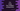# How to swap two numbers using pointer in C++## How to swap two numbers using pointer in C++:

In this post, we will learn how to swap or exchange two numbers using pointer in C++. Pointers are used to keep the address of variables. We will write one function that will take two pointers of two integer variables and swap these integer variables by using the pointers.

Passing pointers to a function is a better way to exchange or change any value because we are passing the memory address of a variable. If we change anything that is stored in that memory address, we don’t have to assign any other value to the variable, it will be changed immediately.

### Algorithm using in this program:

We will use the below algorithm:

• Take the numbers as inputs from the user. Store these numbers in two different int variables.
• Pass the pointers of these variables to a different function.
• Inside the function, exchange the content of these variables using a third variable.

• Keep the content of the second variable in the third variable.
• Keep the content of the first variable in the second variable.
• Keep the content of the third variable in the first variable.
• With these three steps, the values in the variables will be swapped.
• Once done, print the numbers again to the user.

## C++ program:

Below is the complete C++ program that swaps two user input numbers using pointers:

``````#include <iostream>
using namespace std;

void swap(int *first, int *second)
{
int temp;

temp = *first;
*first = *second;
*second = temp;
}

int main()
{
int a, b;

cout << "Enter the numbers : " << endl;
cin >> a;
cin >> b;

cout << "Before swap, a = " << a << ", b = " << b << endl;
swap(&a, &b);
cout << "After swap, a = " << a << ", b = " << b << endl;
}``````

Here,

• a and b are two integer variables.
• We are taking the numbers as inputs from the user and these values are stored in a and b.
• swap is used to swap two number using pointers. It takes two integer pointers as the arguments and swaps the values stored in the addresses pointed by these pointers.

• temp is used to keep the value temporarily.
• It first stores the value of first in temp.
• Then it stores the value of second in first.
• Finally, it stores the value of temp in second. And both numbers are swapped with this step.
• This program is printing the values of the integers before and after the swapping is done.

It will print output as like below:

``````Enter the numbers :
12
13
Before swap, a = 12, b = 13
After swap, a = 13, b = 12``````